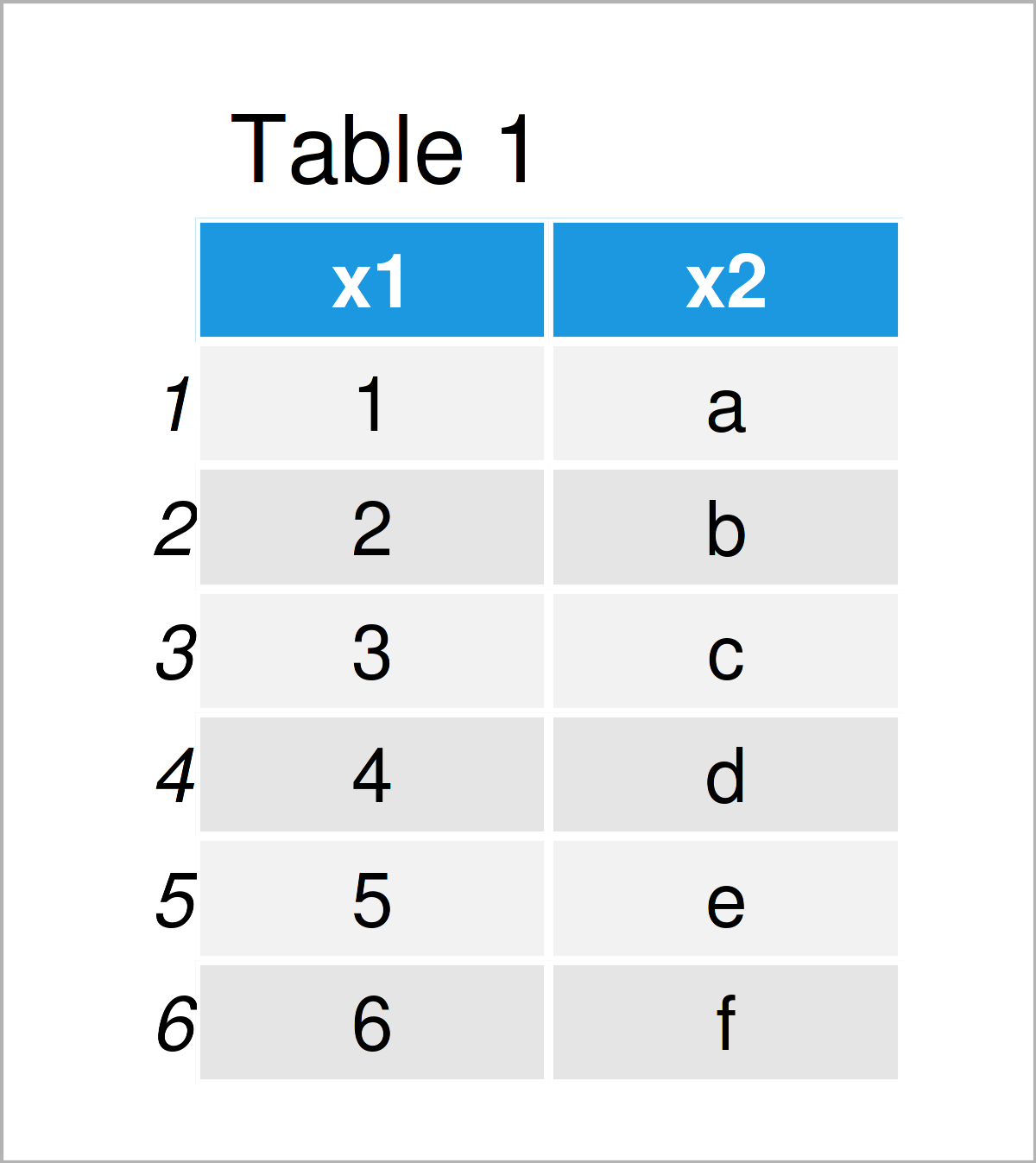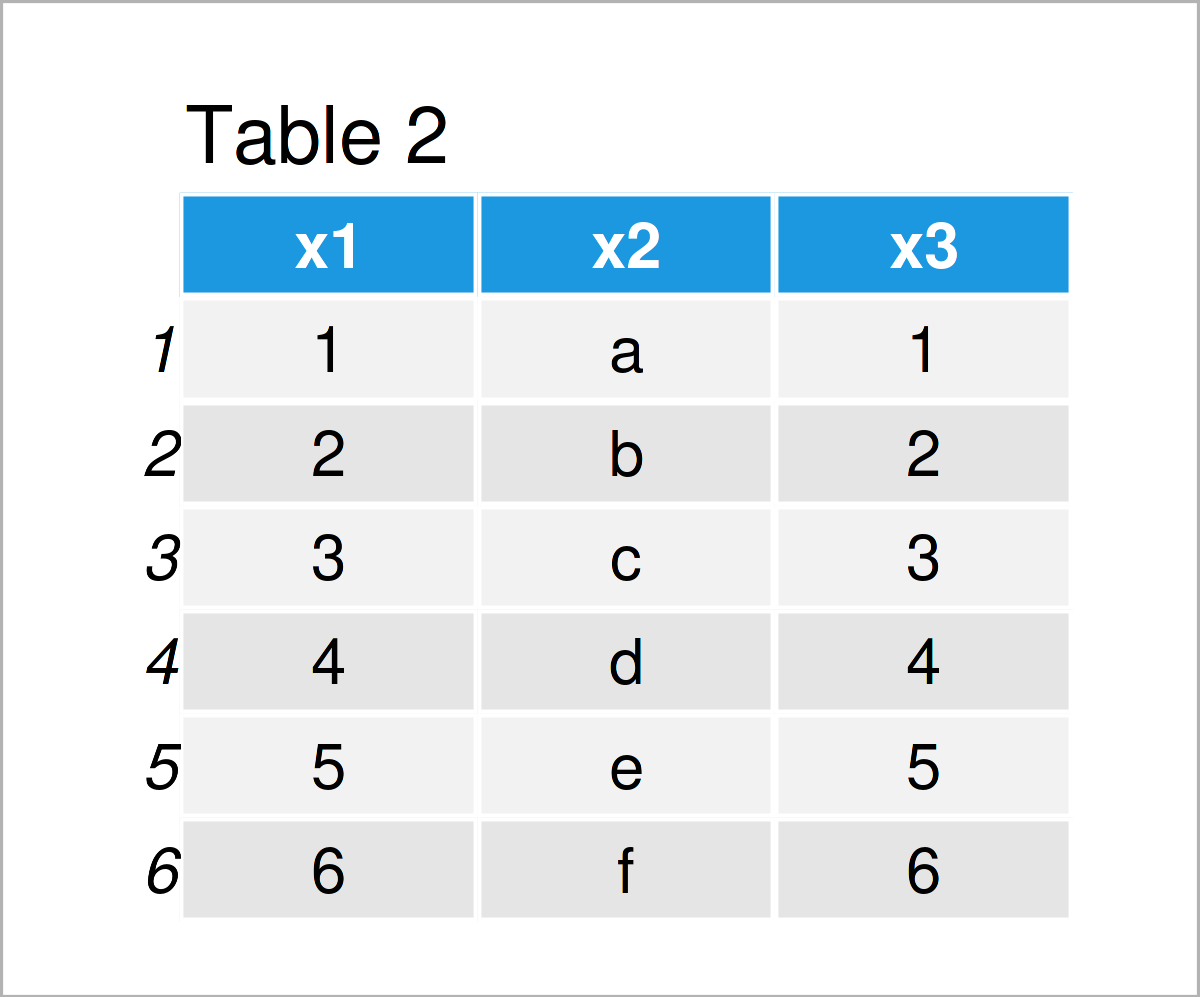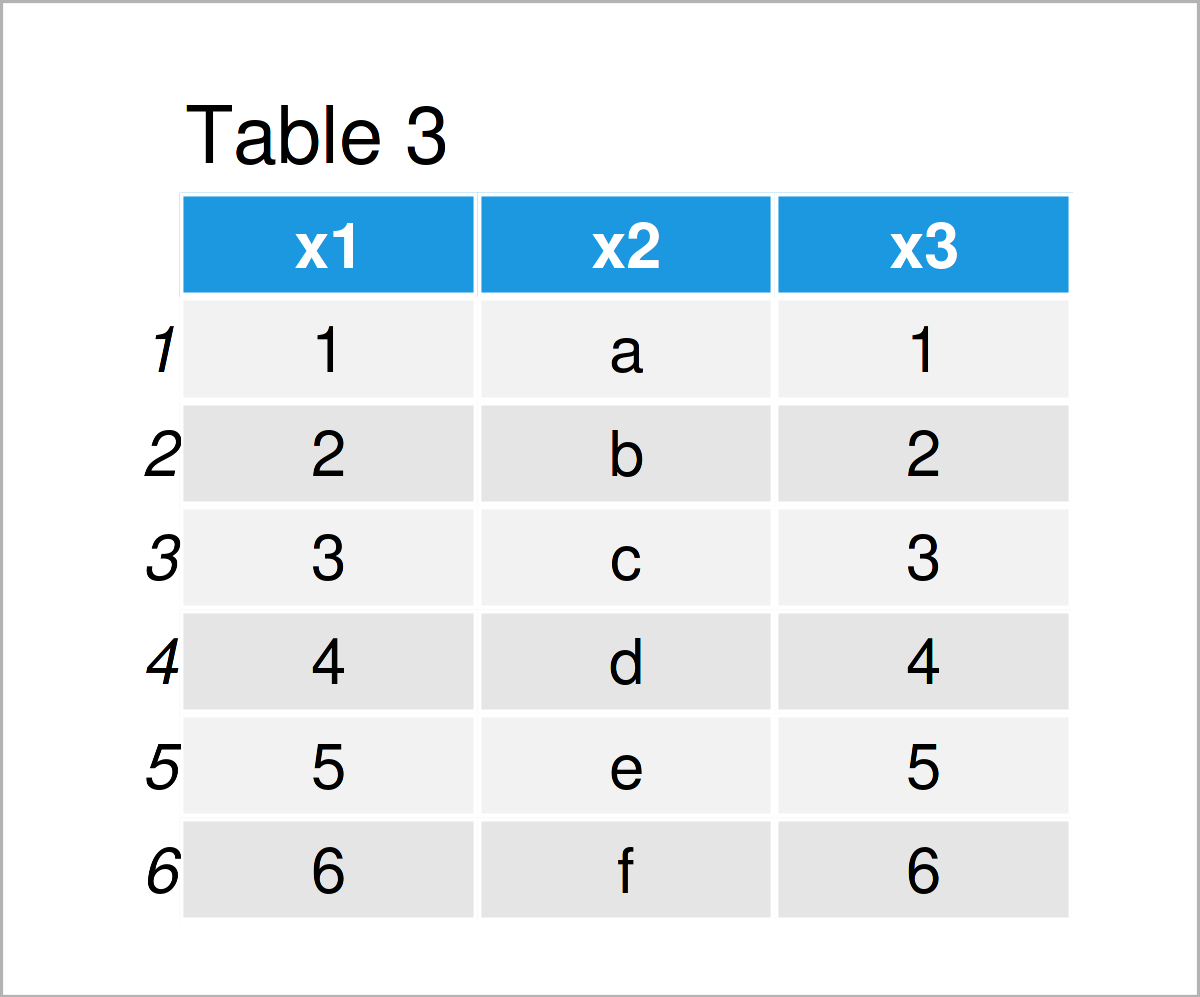# Create Duplicate of Column in R (2 Examples)

In this article you’ll learn how to replicate a column in a data frame in the R programming language.

The article is structured as follows:

It’s time to dive into the examples…

## Exemplifying Data

We’ll use the following data frame as basement for this R tutorial.

```data <- data.frame(x1 = 1:6, # Create example data x2 = letters[1:6]) data # Print example data```As you can see based on Table 1, our example data is a data frame containing six rows and two columns that are named “x1” and “x2”. The column x1 is an integer and the column x2 has the character class.

## Example 1: Create Duplicate of Column Using Base R

The following R code illustrates how to replicate a data frame variable in a data frame with a new name.

Have a look at the following R code and its output:

```data_new1 <- data # Duplicate entire data frame data_new1\$x3 <- data_new1\$x1 # Create duplicate of column with new name data_new1 # Print new data frame```As shown in Table 2, we have created a new data frame called data_new1, which contains a duplicate of the column x1. This duplicate has been called x3.

## Example 2: Create Duplicate of Column Using mutate() Function of dplyr Package

In this example, I’ll explain how to use the functions of the dplyr package to construct a duplicated and renamed variable in a data frame.

We first need to install and load the dplyr package:

```install.packages("dplyr") # Install & load dplyr package library("dplyr")```

Next, we can apply the mutate function to create a replication of the variable x1 as follows:

```data_new2 <- data %>% # Create duplicate of column with new name mutate(x3 = x1) data_new2 # Print new data frame```Table 3 shows the output of the previous syntax – Another data matrix containing the same values as in Example 1. However, this time we have used the dplyr package instead of Base R.

## Video & Further Resources

Have a look at the following video on my YouTube channel. In the video, I illustrate the R programming codes of this tutorial.

In addition, you might want to read the other articles on https://www.statisticsglobe.com/.

This post has illustrated how to duplicate a variable in a data frame in the R programming language. In case you have further questions or comments, let me know in the comments section.

Subscribe to the Statistics Globe Newsletter